# Tank Volume Calculator

By Hanna Pamuła, PhD candidate
Last updated: Oct 05, 2021

With this tank volume calculator, you can easily estimate what the volume of your container is. Choose between nine different tank shapes: from standard rectangular and cylindrical tanks, to capsule and elliptical tanks. You can even find the volume of a frustum in cone bottom tanks. Just enter the dimensions of your container and this tool will calculate the total tank volume for you. You may also provide the fill height, which will be used to find the filled volume. Do you wonder how it does it? Scroll down and you'll find all the formulas needed - the volume of a capsule tank, elliptical tank, or the widely-used cone bottom tanks (sometimes called conical tanks), as well as many more!

Looking for other types of tanks, in different shapes and for other applications? Check out our volume calculator to find the volume of the most common three-dimensional solids. For something more specialized you can also have a glance at the aquarium and pool volume calculators for solutions to everyday volume problems.

## Tank volume calculator

This tank volume calculator is a simple tool which helps you find the volume of the tank as well as the volume of the filled part. You can choose between ten tank shapes:

• vertical cylinder
• horizontal cylinder
• rectangular prism (box)
• vertical capsule
• horizontal capsule
• vertical oval (elliptical)
• horizontal oval (elliptical)
• cone bottom
• cone top
• frustum (truncated cone, funnel-shaped)

"But how do I use this tank volume calculator?", you may be asking. Let's have a look at a simple example:

1. Decide on the shape. Let's assume that we want to find the volume of a vertical capsule tank - choose that option from the drop-down list. The schematic picture of the tank will appear below; make sure it's the one you want!
2. Enter the tank dimensions. In our case, we need to type in the length and diameter. In our example, they are equal to 30 in and 24 in, respectively. Additionally, we can enter the fill height - 32 in.
3. The tank volume calculator has already found the total and filled volume! The total volume of the capsule is 90.09 US gal, and the volume of the liquid inside is 54.84 US gal. As always, you can change the units by clicking on the volume units themselves. Easy-peasy!

## Cylindrical tank volume formula

To calculate the total volume of a cylindrical tank, all we need to know is the cylinder diameter (or radius) and the cylinder height (which may be called length, if it's lying horizontally).

• Vertical cylinder tank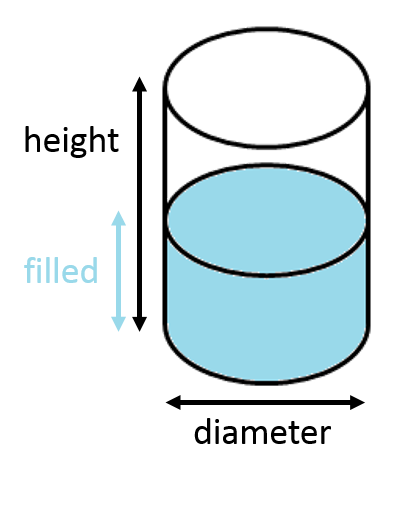The total volume of a cylindrical tank may be found with the standard formula for volume - the area of the base multiplied by height. A circle is the shape of the base, so its area, according to the well-known equation, is equal to `π * radius²`. Therefore the formula for a vertical cylinder tanks volume looks like:

`V_vertical_cylinder = π * radius² * height = π * (diameter/2)² * height `

If we want to calculate the filled volume, we need to find the volume of a "shorter" cylinder - it's that easy!

`V_vertical_cylinder = π * radius² * filled = π * (diameter/2)² * filled `

• Horizontal cylinder tank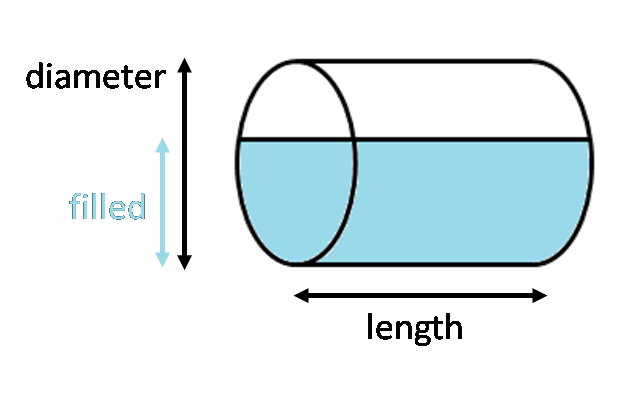The total volume of a horizontal cylindrical tank may be found in analogical way - it's the area of the circular end times the length of the cylinder:

`V_horizontal_cylinder = π * radius² * length = π * (diameter/2)² * length`

Things are getting more complicated when we want to find the volume of the partially filled horizontal cylinder. First, we need to find the base area: the area of the circular segment covered by the liquid:`Segment area = 0.5 * radius² * (θ - sinθ)`

where θ is the central angle of segment, and may be found from the formula for cosine:

`cos(θ/2) = (radius - filled) / radius`

`θ = 2 * arccos((radius - filled) / radius)`

And finally, the formula for partially filled horizontal cylinder is:

`V_horizontal_cylinder_filled = 0.5 * radius² * (θ- sin(θ)) * length` where `θ = 2 * arccos((radius - filled) / radius)`

If the cylinder is more than half full then it's easier to subtract the empty tank part from the total volume.

## Rectangular tank volume calculator (rectangular prism)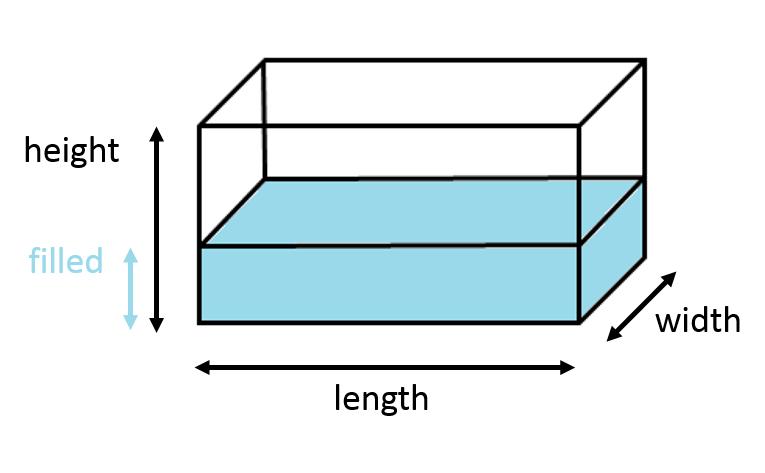If you're wondering how to calculate the volume of a rectangular tank (also known as cuboid, box or rectangular hexahedron), look no further! You may know this tank as a rectangular tank - but that is not its proper name, as a rectangle is a 2D shape, so it doesn't have a volume.

To find the rectangular prism volume, multiply all the dimensions of the tank:

`V_rectangular_prism = height * width * length`

If you want to know what the volume of the liquid in a tank is, simply change the `height` variable into `filled` in the rectangular tank volume formula:

`V_rectangular_prism_filled = filled * width * length`

For this tank volume calculator, it doesn't matter if the tank is in a horizontal or vertical position. Just make sure that `filled` and `height` are along the same axis.

## Formula for volume of a capsule

Our tool defines a capsule as two hemispheres separated by a cylinder. To calculate the total volume of a capsule, all you need to do is add the volume of the sphere to the cylinder part:

`V_capsule = π * (diameter/2)² * ((4/3) * (diameter/2) + length)`

Depending on the position of the tank, the filled volume calculations will differ a bit:

1. For horizontal capsule tank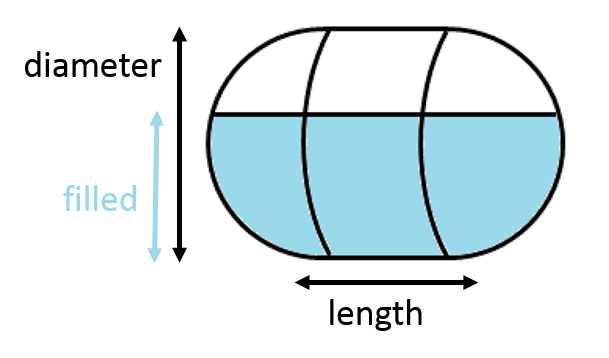As the hemispheres on either end of the tank are identical, they form a spherical cap - add this part to the part from the horizontal cylinder (check the paragraph above) to calculate the volume of the liquid:

`capsule_h_filled = V_horizontal_cylinder_filled + V_spherical_cap_filled = 0.5 * (diameter/2)² * (θ- sin(θ)) * length + ((π * filled²) / 3) * ((1.5 * diameter) - filled)`

2. For vertical capsule tank

The formula differs for various fill heights: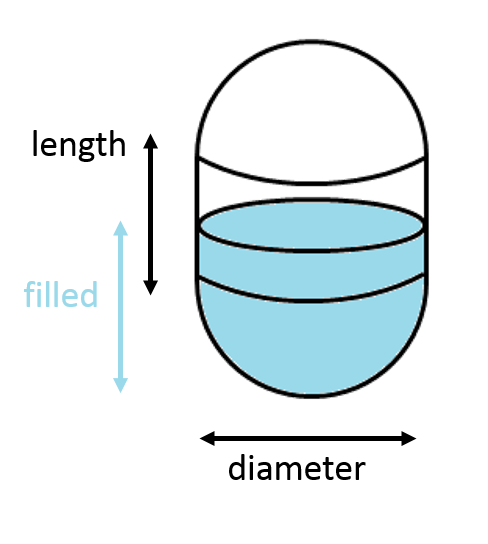• if the filled < diameter/2, then the liquid is only in the bottom hemisphere part, so we only need the volume of a spherical cap formula:

`V_capsule_filled = ((π * filled²) / 3) * ((1.5 * diameter) - filled)`

• if the diameter/2 < filled < diameter/2 + length, then we need to add the hemisphere volume and "shorter" cylinder:

`V_capsule_filled = (2/3) * π * (diameter/2)³ + π * (diameter/2)² * (filled - diameter/2)`

• if the diameter/2 + length < filled, it means that we have full bottom hemisphere and cylinder, so we just need to subtract the spherical cap (empty part) from the whole volume:

`V_capsule_filled = V_capsule - ((π * filled²) / 3) * ((1.5 * diameter) - (length + diameter - filled)))`

## Elliptical tank volume (oval tank)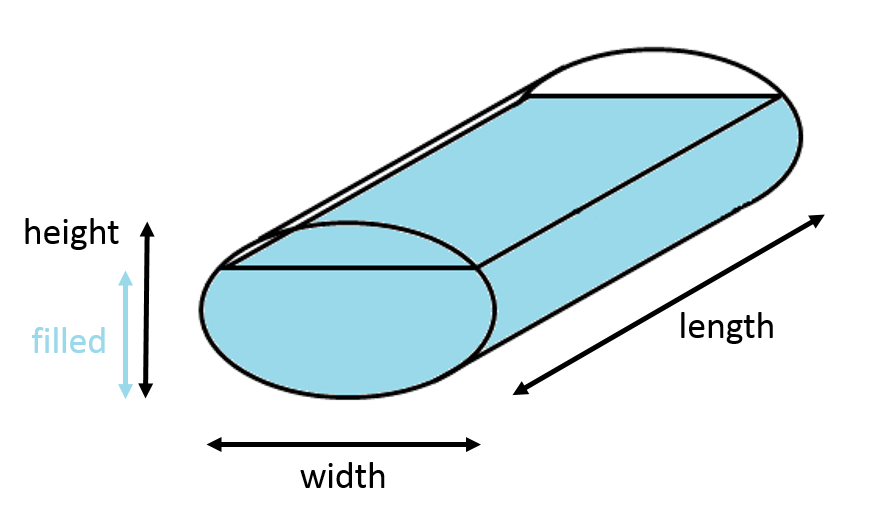In our calculator, we define an oval tank as a cylindrical tank with an elliptical end (not in the shape of a stadium, as it is sometimes defined). To find the total volume of an elliptical tank, you need to multiply the ellipsis area times length of the tank:

`V_ellipse = π * width * length* height / 4`

Finally, another easy formula! Unfortunately, finding the volume of a partially filled tank - both in the horizontal and vertical positions - is not so straightforward. You need to use the formula for the ellipse segment area and multiply the result times length of the tank: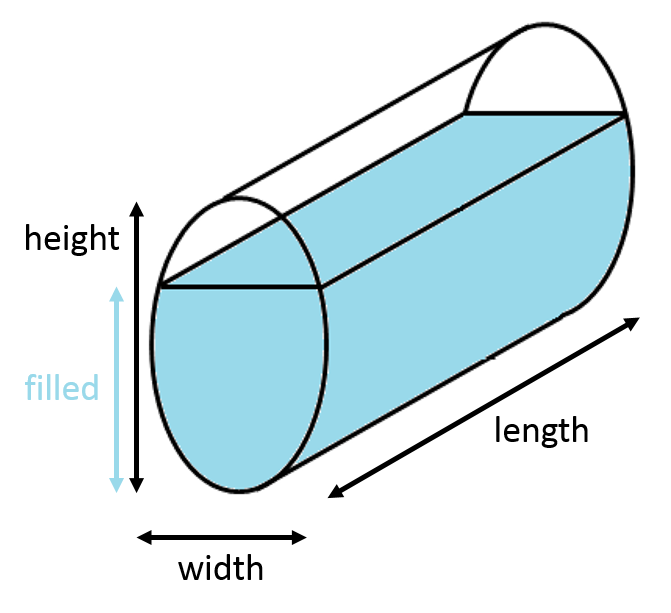`V_ellipse_filled = length * height * width /4 * (arccos(1 - (2 * filled / height)) - (1 - (2 * filled / height)) * √(4 * filled / height - 4 * filled²/height²))`

## Frustum volume - tank in the shape of a truncated cone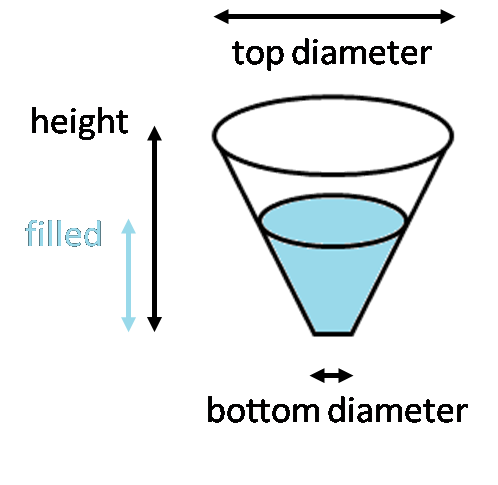To calculate the truncated cone volume, use the formula:

`V_frustum = (1/3) * π * cone_height * ((diameter_top / 2)² + (diameter_top / 2) * (diameter_bottom / 2) + (diameter_bottom / 2)²)`

If you want to find the partially filled frustum volume for a given fill height, calculate the top radius of the filled part first:

`R = 0.5* diameter_top*(filled + z) / (cone_height + z)`where

`z = cone_height * diameter_bottom/(diameter_top - diameter_bottom)`

(You can derive the formula from triangles similarity)

Afterwards, just find the new frustum volume:

`V_frustum_filled = (1/3) * π * cone_height* (R² + R * (diameter_bottom / 2) + (diameter_bottom / 2)²)`

## Cone bottom tank volume (conical tank) and cone top tanks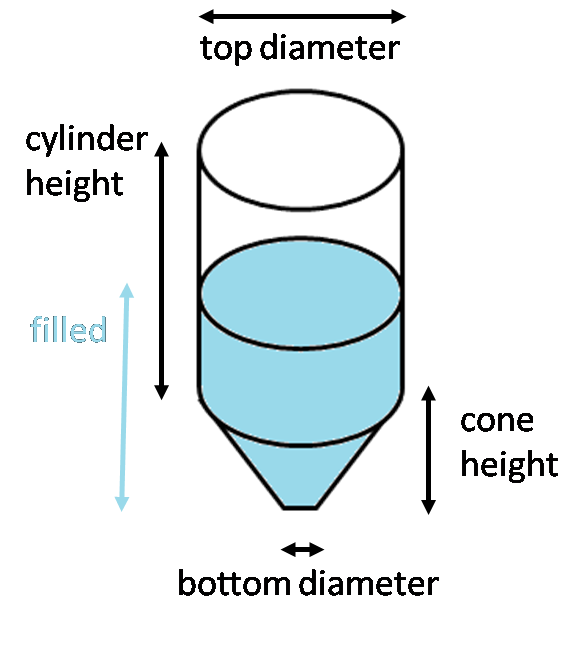Finding total volume of a cone bottom tank is not so hard - just add the volume of the frustum part to the volume of the cylindrical part:

`V_cone_bottom = V_frustum + V_cylinder = (1/3) * π * cone_height * ((diameter_top/2)² + (diameter_top/2)*(diameter_bot/2) + (diameter_bot/2)²) + π *(diameter_top/2)² * cylinder_height`

To calculate the partially filled tank, just add the frustum part and cylinder part, depending on the level of the filled liquid, using the equations above.

Calculating total volume of cone top tank is exactly the same as cone bottom tank. The only difference is when you want to find filled part - of course, firstly the cylindrical part is filled, and only then the frustum.

Hanna Pamuła, PhD candidate
Tank shape
vertical cylinderDimensions
Diameter
ft
Height
ft
Filled
ft
Volume
Total tank volume
US gal
Filled with
US gal
People also viewed…

### Bolt torque

The bolt torque calculator helps you make sure you tighten your bolts correctly.

### Ramp

If you're wondering how to design a ramp, here we come with an amazing ramp calculator.

### Snowman

The perfect snowman calculator uses math & science rules to help you design the snowman of your dreams!

### Sunbathing

Do you always remember to put on sunscreen before going outside? Are you sure that you use enough? The Sunbathing Calculator ☀ will tell you when's the time to go back under an umbrella not to suffer from a sunburn!